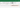# How to refresh and recalculate formulas only in a selected range of cells

When you work with large spreadsheets full of formulas, functions and cell references, it may be frustrating to wait as the spreadsheet is recalculated every time you change a single cell. In fact, it’s likely you have already switched from automatic to manual workbook calculation. And even so, you get only two options: calculate either the entire workbook, or the active worksheet. What is you need to recalculate only one table, or just one column?

The Recalculate Range feature helps do just that:

• Recalculate formulas in selected cells only
• Simply select a range – and click ALT+F9 to refresh
• Or set any custom shortcut you need

## Before you begin, add Recalculate Range to Excel

Recalculate Range is one of the 20+ features within XLTools Add-in for Excel. Works in Excel 2019, 2016, 2013, 2010, desktop Office 365.– free trial gives you 14 days of full access to all features.

## How to switch from automatic to manual calculation in Excel

The Recalculate Range feature will only work if you disable automatic spreadsheet calculation and switch to manual mode:

Open Formulas tabClick Calculation optionsIn the drop-down list, check Manual.

Now automatic calculations of the workbook are disabled. Recalculation of formulas can only be triggered manually, for example through Excel shortcuts.## How to recalculate a specific range in Excel

To recalculate a selected range only, use XLTools shortcut:

Select a rangeUse the keyboard shortcut combination Alt + F9Done! All cells with formulas in the range are recalculated.Note: if your selected range contains formulas, functions, or references that depend on another range, to get correct recalculation results, the other range must be up to date.

To force recalculate an entire workbook or a worksheet, use Excel shortcuts:

• F9 – calculate all worksheets in all open workbooks
• Shift+F9 – calculate the active worksheet
• Ctrl+Alt+F9 – calculate all worksheets in all open workbooks, regardless of whether they have changed since the last calculation
• Ctrl+Alt+Shift+F9 – check dependent formulas, and then calculate all cells in all open workbooks, including cells not marked as needing to be calculated

## How to change default shortcut for the Recalculate Range

You can change the hot key combinations for the Recalculate Range at any time. Note, some shortcuts may not be available if they are reserved for other operations in Excel.

XLTools tabSettings buttonShortcuts tabSet your own shortcutsOK.

Any questions or suggestions?How can we help?